Next: Checkpointing - Grid Images Up: Output Previous: Two Field Histograms

# Slices

The function slices() creates an ASCII file containing the field values at each point in a slice through the lattice. The number slicedim in parameters.h sets the number of dimensions for the slice. There are three other parameters controlling the output of this function, slicelength, sliceskip, and sliceaverage. The first determines how many points (in each dimension) will be included while sliceskip determines how many points will be skipped in between adjacent points on the slice. For example, if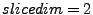,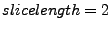, and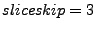then the function will output the lattice points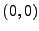,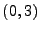,, and. (If the lattice is three dimensional then the first index will be fixed at zero.) If sliceaverage is set to one, however, then all field values in between the array points being sampled will be averaged. In the example above, for instance, the first output value would be the average of the field values at the nine points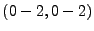.

The file slicetimes simply records the times at which slices were output and the files slicefield_ext record the field values for each output field. The values are recorded in usual C array order, as in the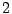xexample just given.Next: Checkpointing - Grid Images Up: Output Previous: Two Field Histograms